GRE Subject Test: Math : Taylor's Theorem

Example Questions

Example Question #132 : Gre Subject Test: Math

For which of the following functions can the Maclaurin series representation be expressed in four or fewer non-zero terms?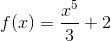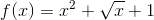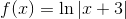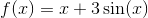Explanation:

Recall the Maclaurin series formula: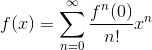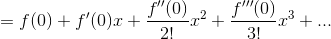Despite being a 5th degree polynomial recall that the Maclaurin series for any polynomial is just the polynomial itself, so this function's Taylor series is identical to itself with two non-zero terms.

The only function that has four or fewer terms isas its Maclaurin series is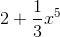.

Example Question #1 : Taylor And Maclaurin Series

Let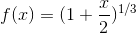Find the the first three terms of the Taylor Series forcentered at.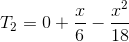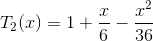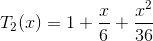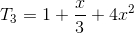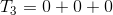Explanation:

Using the formula of a binomial series centered at 0: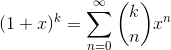,

where we replacewith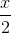and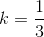, we get: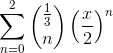for the first 3 terms.

Then, we find the terms where,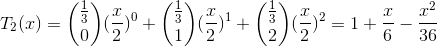Example Question #4 : Applications Of Derivatives

Determine the convergence of the Taylor Series foratwhere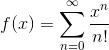.

Conditionally Convergent.

Absolutely Convergent.

Does not exist.

Inconclusive.

Divergent.

Absolutely Convergent.

Explanation:

By the ratio test, the series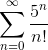converges absolutely: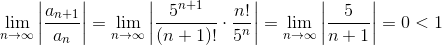Example Question #5 : Applications Of Derivatives

Find the interval of convergence forof the Taylor Series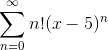.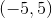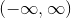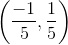Explanation:

Using the root test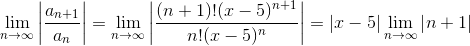and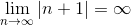. T

herefore, the series only converges when it is equal to zero.

This occurs when x=5.

Example Question #6 : Applications Of Derivatives

Suppose that the derivative of a function, denoted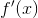, can be approximated by the third degree Taylor polynomial, centered at: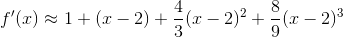If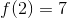, find the third degree Taylor polynomial forcentered at.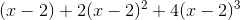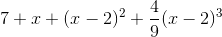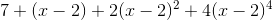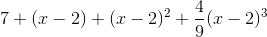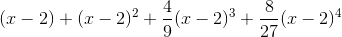Explanation:

To get, we need to find the antiderivative ofby integrating the third degree polynomial term by term.

We only want up to a third degree polynomial, so we can disregard the fourth order term: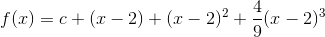Since, substitute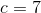for the final.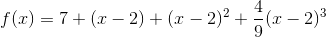Example Question #1 : Taylor's Theorem

Write out the first four terms of the Taylor series about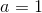for the following function: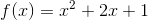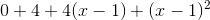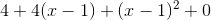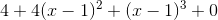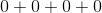Explanation:

The Taylor series about x=a of any function is given by the following: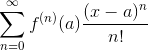So, we must find the zeroth, first, second, and third derivatives of the function (for n=0, 1, 2, and 3 which makes the first four terms):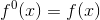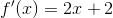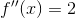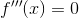The derivatives were found using the following rule: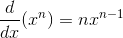Now, evaluated at x=a=1, and plugging in the correct n where appropriate, we get the following: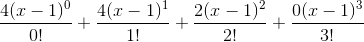which when simplified is equal to.

Example Question #2 : Taylor's Theorem

Find the first two terms of the Taylor series about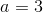for the following function: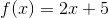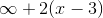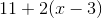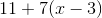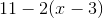Explanation:

The general formula for the Taylor series about x=a for a function isFirst, we must find the zeroth and first derivative of the function.

The zeroth derivative of a function is just the function itself, so we only have to find the first derivative: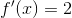The derivative was found using the following rule:Now, write the first two terms of the sequence (n=0 and n=1):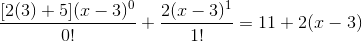Example Question #11 : Applications Of Derivatives

Write out the first three terms of the Taylor series for the following function about: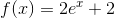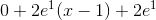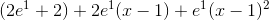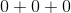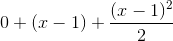Explanation:

The general formula for the Taylor series of a given function about x=a is.

We were asked to find the first three terms, which correspond to n=0, 1, and 2. So first, we need to find the zeroth, first, and second derivative of the given function. The zeroth derivative is just the function itself.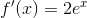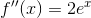The derivatives were found using the following rules: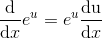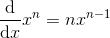Now use the above formula to write out the first three terms: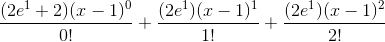Simplified, this becomesAll GRE Subject Test: Math Resources